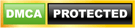# Answer -Question of the Week – 25/12/2013- Question 1

Question:- Suppose we have a table says tbl_students whose structure is given below:-

Create table tbl_students(Studentid int identity(1,1) , Studentname nvarchar(150))

Suppose it contains the following data:-

Studentid  Studentname
1               Vivek Johari
2               Chandra Singh
4               Avinash Dubey
6               Akhil
7               Sunny Rana
10             Saurabh

As one can see from the data that some rows are deleted from the table as some studentids are missing.

So write a query which gives the studentid which are deleted from this table

Thanks all for answering that question. I really appreciate the effort of all the people who tried and gives the response.We get multiple answers for this question in which correct answer is given below:-

Correct answer in case the first coulmn is an identity column

WITH Numbers AS (
SELECT 1 AS Number
UNION ALL
SELECT Number + 1
FROM Numbers
WHERE Number < IDENT_CURRENT(‘tbl_students’)
)
SELECT Number
FROM Numbers N
LEFT JOIN tbl_students S ON N.Number = S.StudentId
WHERE S.StudentId IS NULL

Answer given By Chandra Prakash Singh

select number from master..spt_values s
left join tbl_students on s.number = tbl_students.studentid
where s.type=’P’
and s.number <= IDENT_CURRENT(‘tbl_students’)
and tbl_students.studentid is null and number > 0

Correct answer in case the first coulmn is not an identity column

WITH DeletedRows (missnum, maxid)
AS

SELECT 1 AS missnum, (select max(Studentid) from tbl_Students) UNION ALL SELECT missnum + 1, maxid FROM DeletedRows
WHERE missnum < maxid
)
SELECT missnum FROM DeletedRows
LEFT OUTER JOIN tbl_Students e on e.Studentid = DeletedRows.missnum
WHERE e.Studentid is NULL OPTION (MAXRECURSION 0)

select number from master..spt_values s
left join tbl_students on s.number = tbl_students.studentid
where s.type=’P’
and s.number < (select MAX(studentid) from tbl_students)
and tbl_students.studentid is null and number > 0

Also I appreciate the effort done by chetan Deshpande in attempting the question

## 27 thoughts on “Answer -Question of the Week – 25/12/2013- Question 1”

1.Vimal Kumar Prajapati says:

Is this correct??

BEGIN TRAN
DECLARE @MAX INT= (SELECT MAX(Studentid) FROM TBL_STUDENTS)
CREATE TABLE #T1(ID INT)
WHILE(@MAX>0) BEGIN
INSERT INTO #T1 VALUES(@MAX)
SET @MAX=@MAX-1
END
SELECT * FROM #T1 except (SELECT Studentid FROM TBL_STUDENTS)
DROP TABLE #T1
COMMIT TRAN

2. Pingback: xembongdatructuyen
3. Pingback: w88
4. Pingback: nha cai fun88
5. Pingback: playerqq
6. Pingback: i99 casino
7. Pingback: m88sb
8. Pingback: idrpokerq
9. Pingback: fun88.com
10. Pingback: qiuqiu 99
11. Pingback: vào m88
12. Pingback: hondaqq
13. Pingback: main qq